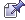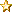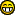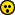Default Skins: Default | Black | Red | Green | Brown | Lavender

Techno Skins: Black | Blue | Brown | Green | Orange | Pink | Purlple | Red | Turquoise

Light Skins: Blue | Brown | Golden | Lilac | Navy | Olive | Red | Rose | Silver | Turqouise | VioletSeptember 28, 2023, 04:38:07 pm
 News:# Mathamatic Formulas And Additional Help.

 Pages:Author Topic: Mathamatic Formulas And Additional Help.  (Read 887 times)
Revival Jam
Community Member
MemberRep: +132/-1
SG:Posts: 63« on: June 10, 2008, 02:32:29 am »

Mathamatic Formulas

Exponential Identities

Powers
x a x b = x (a + b)
x a y a = (xy) a

(x a) b = x (ab)

x (a/b) = bth root of (x a) = ( bth (x) ) a

x (-a) = 1 / x a

x (a - b) = x a / x b
______________________________________________
Logarithms

y = logb(x) if and only if x=b y
logb(1) = 0

logb(b) = 1

logb(x*y) = logb(x) + logb(y)

logb(x/y) = logb(x) - logb(y)

logb(x n) = n logb(x)

logb(x) = logb(c) * logc(x) = logc(x) / logc(b)

________________________________________________

Interest and Exponential Growth

The Compound Interest Equation

P = C (1 + r/n) nt

where
P = future value
C = initial deposit
r = interest rate (expressed as a fraction: eg. 0.06)
n = # of times per year interest in compounded
t = number of years invested

Simplified Compound Interest Equation

When interest is only compounded once per yer (n=1), the equation simplifies to:

P = C (1 + r) t

Continuous Compound Interest
When interest is compounded continually (i.e. n --> ), the compound interest equation takes the form:
P = C e rt

Demonstration of Various Compounding
The following table shows the final principal (P), after t = 1 year, of an account initally with C = \$10000, at 6% interest rate, with the given compounding (n). As is shown, the method of compounding has little effect. n P
1 (yearly) \$ 10600.00
2 (semi-anually) \$ 10609.00
4 (quarterly) \$ 10613.64
12 (monthly) \$ 10616.78
52 (weekly) \$ 10618.00
365 (daily) \$ 10618.31
continuous \$ 10618.37

Loan Balance
Situation: A person initially borrows an amount A and in return agrees to make n repayments per year, each of an amount P. While the person is repaying the loan, interest is accumulating at an annual percentage rate of r, and this interest is compounded n times a year (along with each payment). Therefore, the person must continue paying these installments of amount P until the original amount and any accumulated interest is repayed. This equation gives the amount B that the person still needs to repay after t years.
B = A (1 + r/n)nt - P  (1 + r/n)nt - 1
--------------------------------------------------------------------------------
(1 + r/n) - 1

where
B = balance after t years
A = amount borrowed
n = number of payments per year
P = amount paid per payment
r = annual percentage rate (APR)

Multiplication Tablex 0 1 2 3 4 5 6 7 8 9 10 11 12
0 0 0 0 0 0 0 0 0 0 0 0 0 0
1 0 1 2 3 4 5 6 7 8 9 10 11 12
2 0 2 4 6 8 10 12 14 16 18 20 22 24
3 0 3 6 9 12 15 18 21 24 27 30 33 36
4 0 4 8 12 16 20 24 28 32 36 40 44 48
5 0 5 10 15 20 25 30 35 40 45 50 55 60
6 0 6 12 18 24 30 36 42 48 54 60 66 72
7 0 7 14 21 28 35 42 49 56 63 70 77 84
8 0 8 16 24 32 40 48 56 64 72 80 88 96
9 0 9 18 27 36 45 54 63 72 81 90 99 108
10 0 10 20 30 40 50 60 70 80 90 100 110 120
11 0 11 22 33 44 55 66 77 88 99 110 121 132
12 0 12 24 36 48 60 72 84 96 108 120 132 144

Remembering 9's
What's 9 x 7 ? Use the 9-method! Hold out all 10 fingers, and lower the 7th finger. There are 6 fingers to the left and 3 fingers on the right.

If there is anything else,or forumals,please PM me.

 « Last Edit: June 10, 2008, 02:33:06 am by Supernovadelta » Report SpamLogged

Staff
Sr. MemberRep: +200/-1
Posts: 465« Reply #1 on: June 10, 2008, 02:45:41 am »

In the
Code:
Logarithms

y = logb(x) if and only if x=b y
logb(1) = 0

logb(b) = 1

logb(x*y) = logb(x) + logb(y)

logb(x/y) = logb(x) - logb(y)

logb(x n) = n logb(x)

logb(x) = logb(c) * logc(x) = logc(x) / logc(b)

The logb,can also be changed in refrence to logb(p x) = n lopx (np)
The formula here tells you that the addition complexcity is defined as multiplication not devision.
 Report SpamLogged

Code:
Supernovadelta spots anybody spamming,he will send the Terminater to their house.Revival Jam
Community Member
MemberRep: +132/-1
SG:Posts: 63« Reply #2 on: June 10, 2008, 02:50:33 am »

In the
Code:
Logarithms

y = logb(x) if and only if x=b y
logb(1) = 0

logb(b) = 1

logb(x*y) = logb(x) + logb(y)

logb(x/y) = logb(x) - logb(y)

logb(x n) = n logb(x)

logb(x) = logb(c) * logc(x) = logc(x) / logc(b)

The logb,can also be changed in refrence to logb(p x) = n lopx (np)
The formula here tells you that the addition complexcity is defined as multiplication not devision.

In some ways yes,and you can always change the (PX) to any variable in the equation.
 Report SpamLogged
Ghost Rider
V++ Helper
Sr. MemberRep: +42/-1
SG:Posts: 117« Reply #3 on: June 10, 2008, 03:12:07 am »

This looks realy hard.
 Report SpamLogged

V++ editor and coder.
Y8razer
First Time PosterRep: +1/-0
Posts: 2« Reply #4 on: June 11, 2008, 12:40:00 am »

Looks nice and clean,good job!Report SpamLogged
Staff
Sr. MemberRep: +200/-1
Posts: 465« Reply #5 on: June 11, 2008, 01:59:48 am »

This looks realy hard.

Not realy,if you know your algebra.
 Report SpamLogged

Code:
Supernovadelta spots anybody spamming,he will send the Terminater to their house.History
IRC Developer
Community Member
Junior MemberRep: +18/-0
SG:Posts: 20« Reply #6 on: June 20, 2008, 08:52:10 pm »

This looks realy hard.

Not realy,if you know your algebra.
I agree,you got to know your algebra.
 Report SpamLogged

IRC
Revival Jam
Community Member
MemberRep: +132/-1
SG:Posts: 63« Reply #7 on: August 09, 2008, 12:39:23 am »

This looks realy hard.

Not realy,if you know your algebra.
I agree,you got to know your algebra.
If you want to succeed in life,I'll have to agree with you people.
 Report SpamLogged
thepure_ofswizy
First Time PosterRep: +0/-0
Posts: 4« Reply #8 on: January 27, 2010, 06:01:40 pm »

lol whats with the multiplication tableReport SpamLogged
 Pages: 

Elaborate Search Engine

 Directories Yahoo DMOZ Snap Search Engines Alta Vista Direct Hit Lycos Excite Webcrawler Go.com DejaNews Google Meta Searches Go2Net Search.Com Mamma DogPile

Enter text to find:

AquaAngular was created by deathwilldie of Ultimate GraphicsShoutbox [Open]

Fill your Birth-Date in in the below fields

Month Day Year Hour

This is how long you live:

 Months : Weeks : Days : Hours : Minutes : Seconds : Milliseconds :